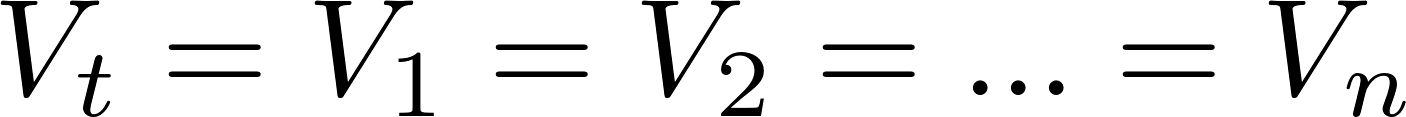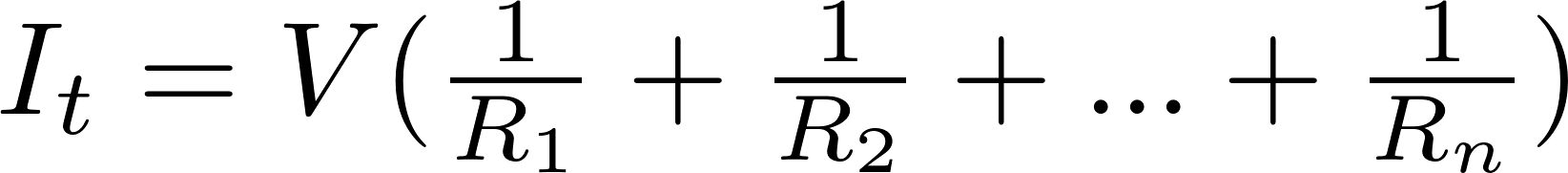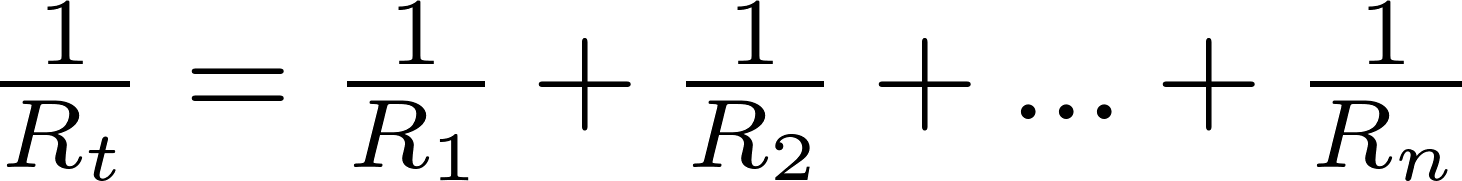# Resistors in parallel

Resistors can be connected in parallel to decrease the total resistance. The resistance is equal to the reciprocal (inverse) of the sum of the reciprocal of all individual resistances. The voltage of all resistors is the same. The total current can be calculated using Ohm's law and is equal to the voltage divided by the total resistance.

### Formulas

The total voltage, current, and resistance are calculated like this: